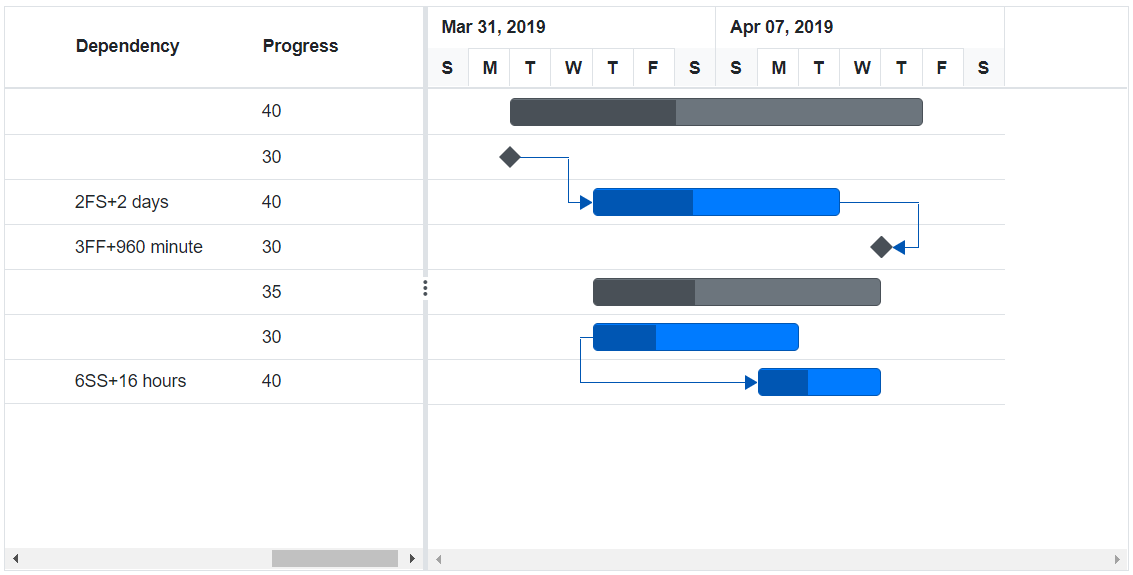Search results

# Task Dependencies in Blazor Gantt Chart component

Task dependency or task relationship can be established between two tasks in Gantt Chart. This dependency affects the project schedule. If you change the predecessor of a task, it will affect the successor task, which will affect the next task, and so on.

Task relationships are categorized into four types based on the start and finish dates of the task.

### Start to Start (SS)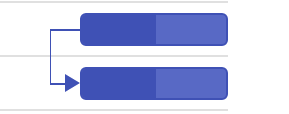### Start to Finish (SF)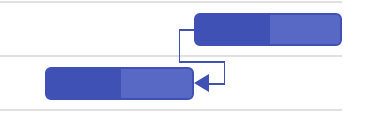### Finish to Start (FS)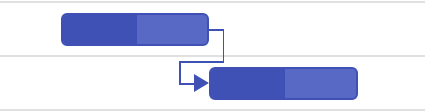### Finish to Finish (FF)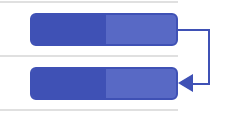Task relationship is defined in the data source as a string value, and this value is mapped to the Gantt Chart component by using the `TaskFields.Dependency` property. The following code example demonstrates how to enable the predecessor in the Gantt Chart component.

``````@using Syncfusion.Blazor.Gantt
</SfGantt>

@code{
protected override void OnInitialized()
{
}

{
public int TaskId { get; set; }
public string TaskName { get; set; }
public DateTime StartDate { get; set; }
public DateTime EndDate { get; set; }
public string Duration { get; set; }
public int Progress { get; set; }
public string Predecessor { get; set; }
}

StartDate = new DateTime(2019, 04, 02),
EndDate = new DateTime(2019, 04, 21),
StartDate = new DateTime(2019, 04, 02),
Duration = "0",
Progress = 30,
},
StartDate = new DateTime(2019, 04, 02),
Duration = "4",
Progress = 40,
Predecessor = "2"
},
StartDate = new DateTime(2019, 04, 02),
Duration = "0",
Progress = 30,
Predecessor = "3"
},
})
},
StartDate = new DateTime(2019, 04, 02),
EndDate = new DateTime(2019, 04, 21),
TaskName = "Develop floor plan for estimation",
StartDate = new DateTime(2019, 04, 04),
Duration = "3",
Progress = 30,
Predecessor = "4"
},
StartDate = new DateTime(2019, 04, 04),
Duration = "3",
Progress = 40,
Predecessor = "6"
},
StartDate = new DateTime(2019, 04, 04),
Duration = "0",
Progress = 30,
Predecessor = "7"
},
})
}
};

}
}``````

The following screenshot displays the output of the above code.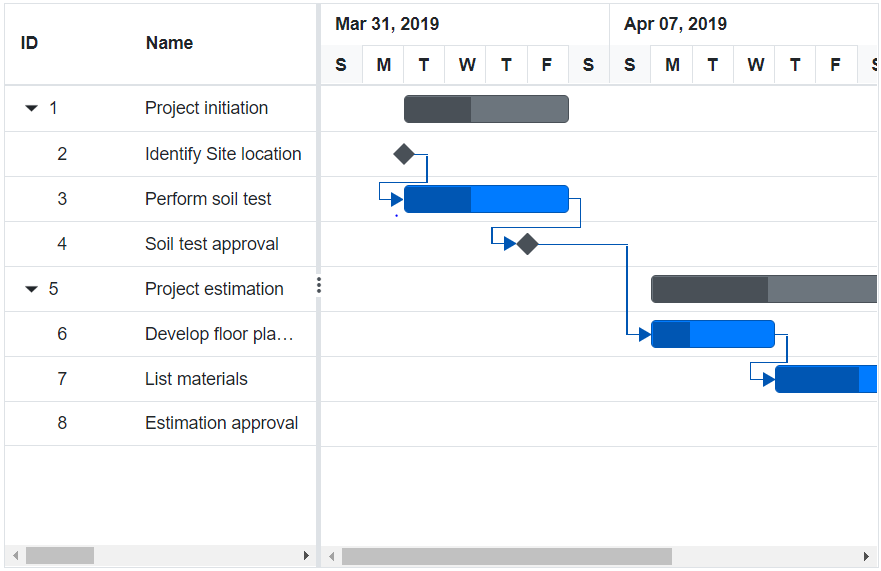## Predecessor offset with duration units

In the Gantt Chart component, the predecessor offset can be defined with the following duration units:

• Day
• Hour
• Minute

You can define an offset with various offset duration units for predecessors by using the following code example.

``````@using Syncfusion.Blazor.Gantt
</SfGantt>
@code{
protected override void OnInitialized()
{
}

{
public int TaskId { get; set; }
public string TaskName { get; set; }
public DateTime StartDate { get; set; }
public DateTime EndDate { get; set; }
public string Duration { get; set; }
public int Progress { get; set; }
public string Predecessor { get; set; }
}

StartDate = new DateTime(2019, 04, 02),
EndDate = new DateTime(2019, 04, 21),
StartDate = new DateTime(2019, 04, 02),
Duration = "0",
Progress = 30,
},
StartDate = new DateTime(2019, 04, 02),
Duration = "4",
Progress = 40,
Predecessor = "2FS+2d"
},
StartDate = new DateTime(2019, 04, 02),
Duration = "0",
Progress = 30,
Predecessor = "3FF+960m"
},
})
},
StartDate = new DateTime(2019, 04, 02),
EndDate = new DateTime(2019, 04, 21),
TaskName = "Develop floor plan for estimation",
StartDate = new DateTime(2019, 04, 04),
Duration = "3",
Progress = 30,
},
StartDate = new DateTime(2019, 04, 04),
Duration = "3",
Progress = 40,
Predecessor = "6SS+16h"
},
})
}
};